# GRE Math : How to find the number of integers between two other integers

## Example Questions

### Example Question #1 : How To Find The Number Of Integers Between Two Other Integers

x = the total number of positive, odd 2 digit numbers less than 100

Column A

x

Column B

45

Possible Answers:

The relationship cannot be determined from the given information

The two quantities are equal

The quantity in column B is greater

The quantity in column A is greater

Correct answer:

The two quantities are equal

Explanation:

There are 50 positive, odd numbers less than 100, and 45 of them are 2 digit numbers.

### Example Question #1 : How To Find The Number Of Integers Between Two Other Integers

0 < x < y < z < 10

x, y, and z are integers.

Quantity A: –7

Quantity B: x + y – z

Possible Answers:

Quantity A is greater.

The two quantities are equal.

Quantity B is greater.

The relationship cannot be determined from the information given.

Correct answer:

Quantity B is greater.

Explanation:

Since:

1. There are only 9 integers between 0 and 10
2. x, y, and z must all be unique
3. They must be specifically ordered such that x < y < z

There are actually not too many ways in which these numbers can be chosen. So what we can do is find a range of answers for Quantity B, and see if 6 falls a) below b) above or c) in between the range.

For the maximum:

Note that the term (x + y) is maximized when x and y are maximum. The (–z) term is maximized when z is minimized. However, there are 2 terms in (x + y) and one term in (–z); thus intuitively it seems we should prioritize (x+y). To make x and y maximum:

0 < 7 < 8 < 9 < 10 since x, y, and z must be unique.

Thus maximum: (x + y – z) = 7 + 8 – 9 = 6

For the minimum:

Note that (x + y) is minimum when (x) and (y) are minimum, and (–z) is minimum when (z) itself is maximimized. However since there are 2 terms in (x+y) and1 of (–z) , again intuititively you should prioritize  (x+y) over (-z). Then in order to make this the least number possible, x and y would be:

min(x + y – z) = 1 + 2 – 9 = –6

Thus, the range of possible answers is:

(x + y – z): [–6, 6]

and –7 is always less than this amount.

### Example Question #1 : How To Find The Number Of Integers Between Two Other Integers

Quantity A: The number of positive even integers less than 1000

Quantity B: The number of positive odd integers less than 1000

Possible Answers:

Quantity A is greater.

Quantity B is greater.

The relationship cannot be determined from the information given.

The two quantities are equal.

Correct answer:

Quantity B is greater.

Explanation:

The question asks for the number of positive even and odd integers less than 1000. Because 1000 is not included, the numbers to consider are 1 through 999. Every positive odd integer will have a corresponding even integer (1 and 2, 3 and 4, 5 and 6, etc.) until you get to 999. This gives the positive odd integers one more number than the number of positive even integers.

### Example Question #2 : How To Find The Number Of Integers Between Two Other Integers

Miles is 3 years older than Ashley. Ashley is 5 years younger than Bill. How old is Ashley if together the three of their ages sum to 44?

Possible Answers:

12

16

10

8

14

Correct answer:

12

Explanation:

Miles is 3 years older than Ashley, so M = A + 3. Also, Bill is 5 years older than Ashley, so B = A + 5.  Together the three of their ages sum to 44, thus:

A + A + 3 + A + 5 = 44

3A + 8 = 44

3A = 36

A = 12

### Example Question #2 : How To Find The Number Of Integers Between Two Other Integers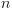is a positive integer between 200 and 500

Quantity A: The number of possible values ofwith a units digit of 5

Quantity B: 31

Possible Answers:

Quantity A is greater

Quantity B is greater

The information cannot be determined from the information given.

The two quantities are equal

Correct answer:

Quantity B is greater

Explanation:

An integer with a units digit of 5 occurs once every 10 consecutive integers. There are 300 integers between 200 and 500, so there must be 30 values with a units digit of 5.

### Example Question #1 : How To Find The Number Of Integers Between Two Other Integers

In a certain game, integers are called magic numbers if they are multiples of eitheror.

How many magic numbers are there in the game between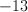and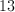?

Possible Answers: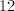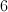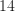Correct answer:Explanation:

There are 13 "magic" numbers: 3,4,6,8,9,12, their negative counterparts, and 0.

### All GRE Math Resources